Successfully reported this slideshow.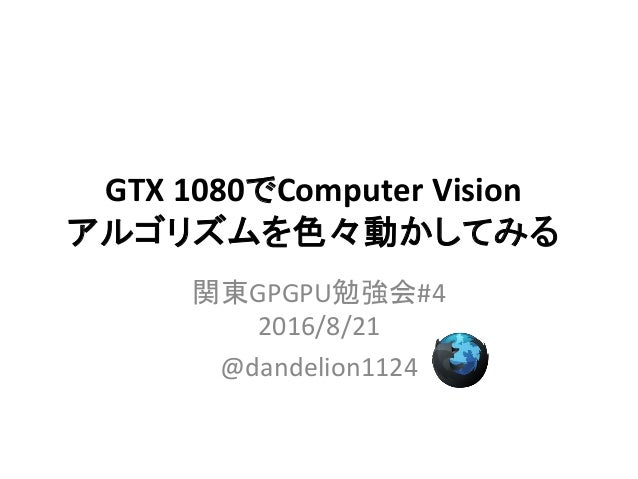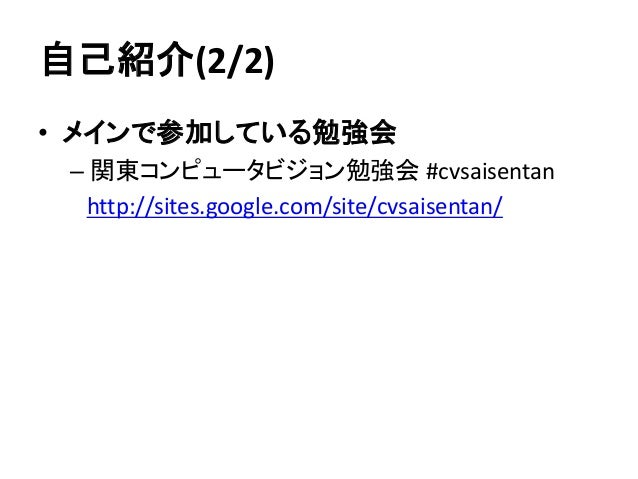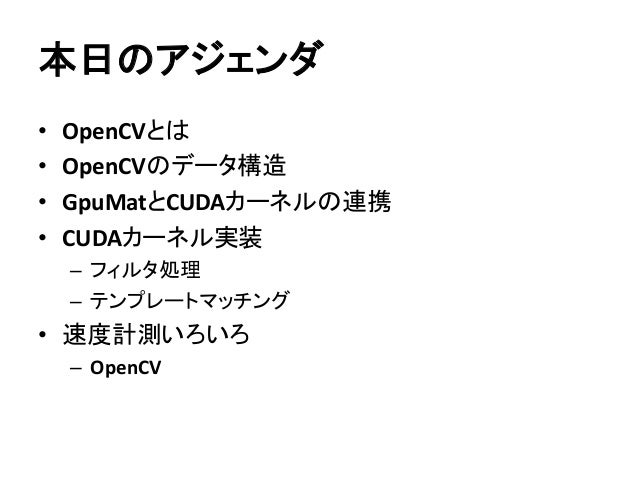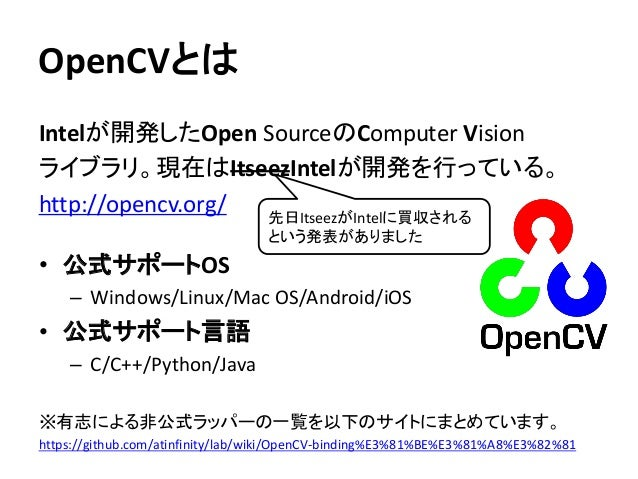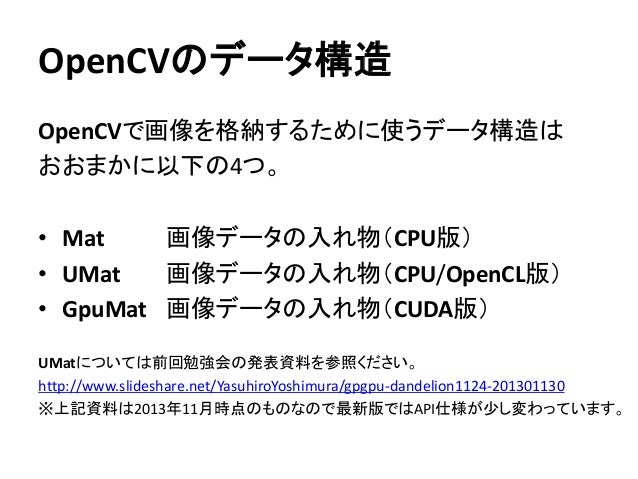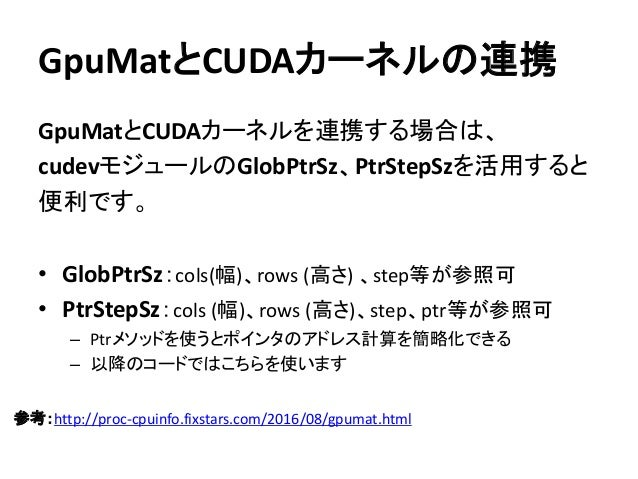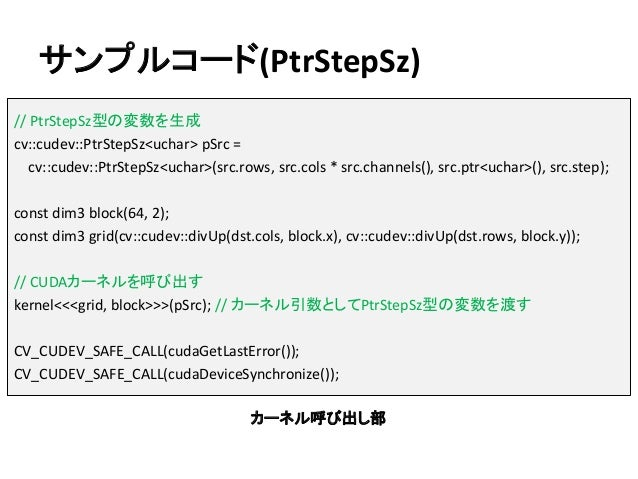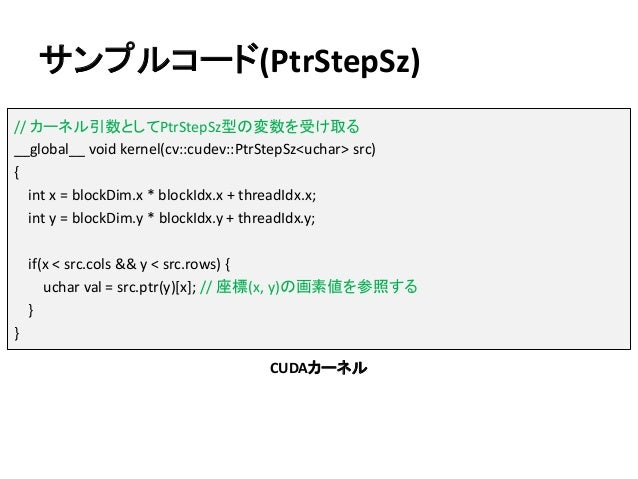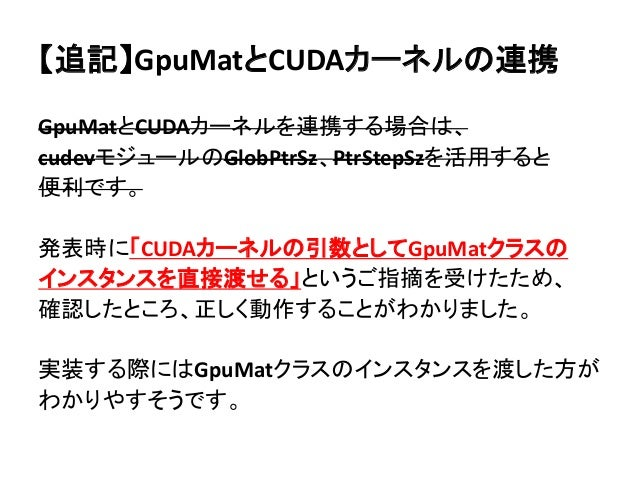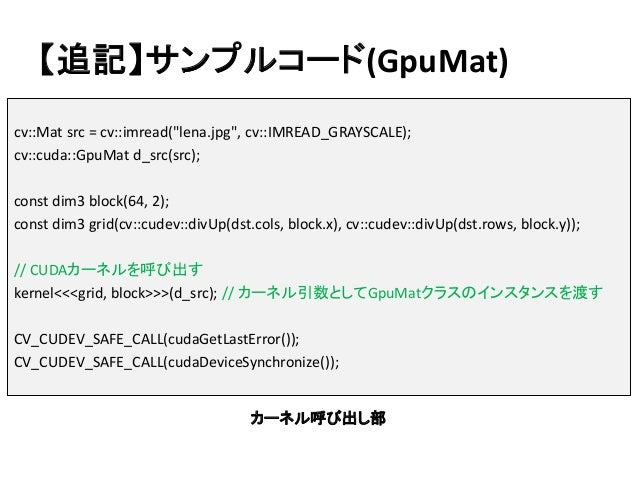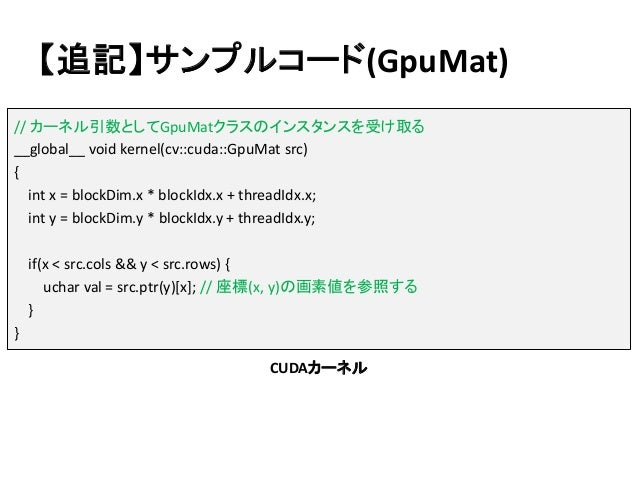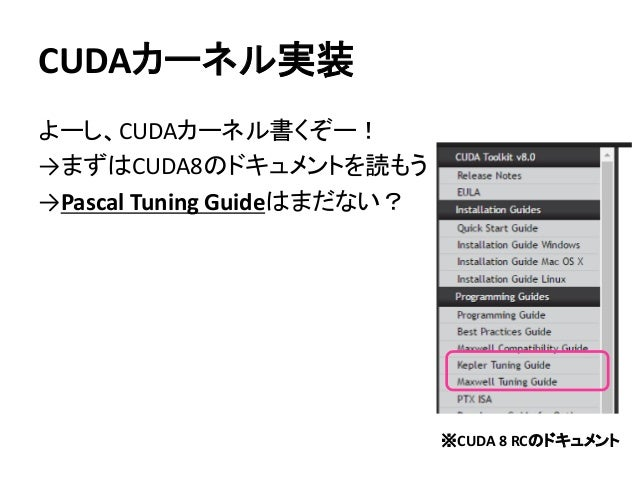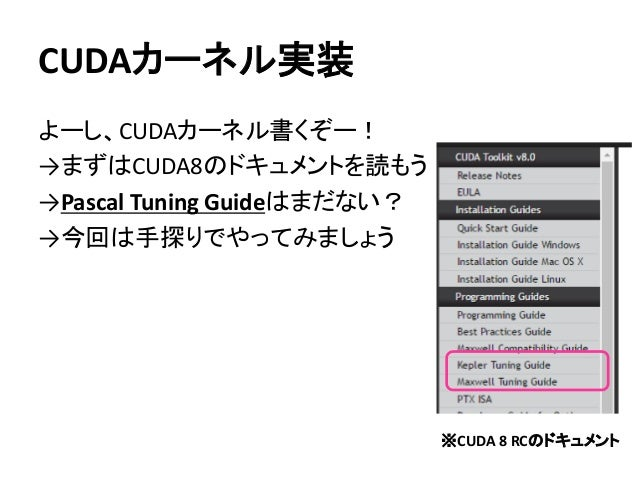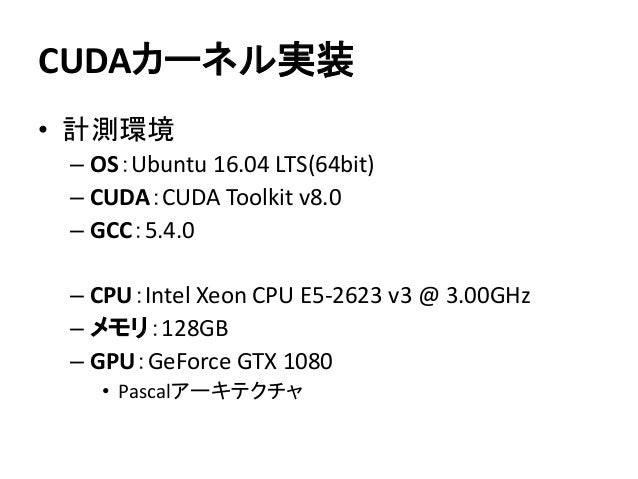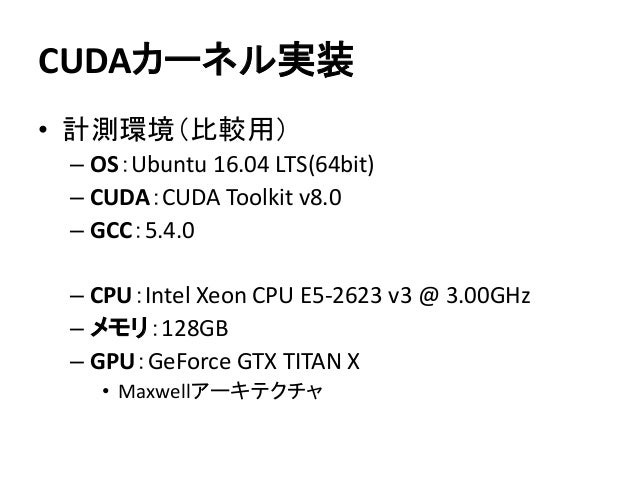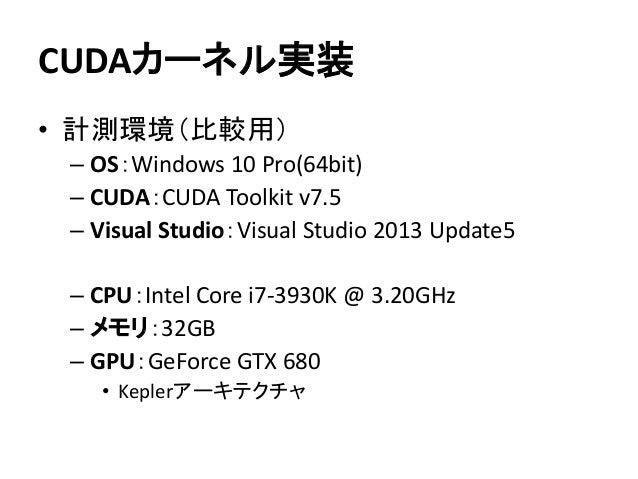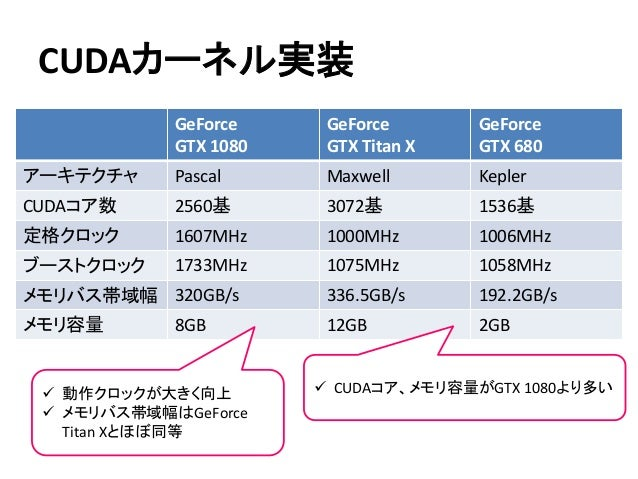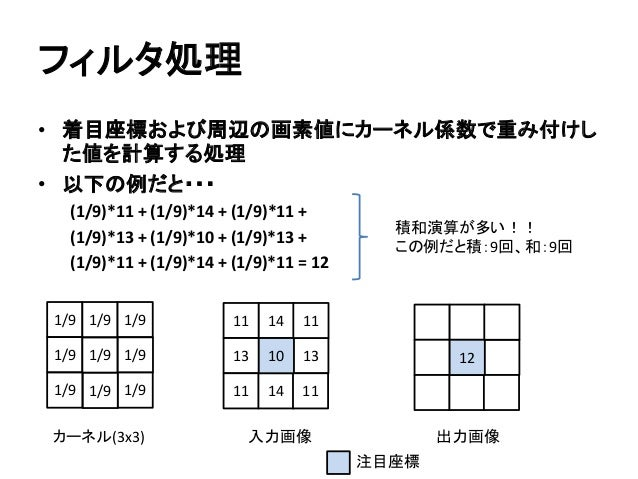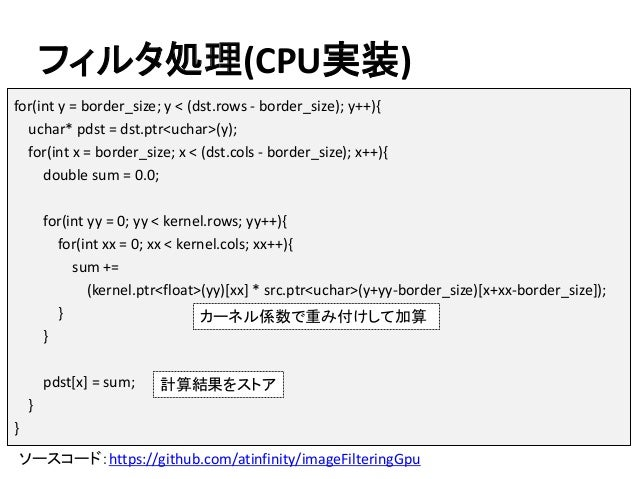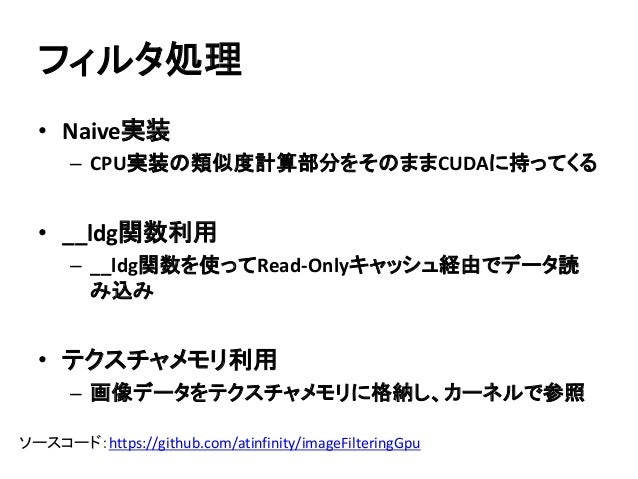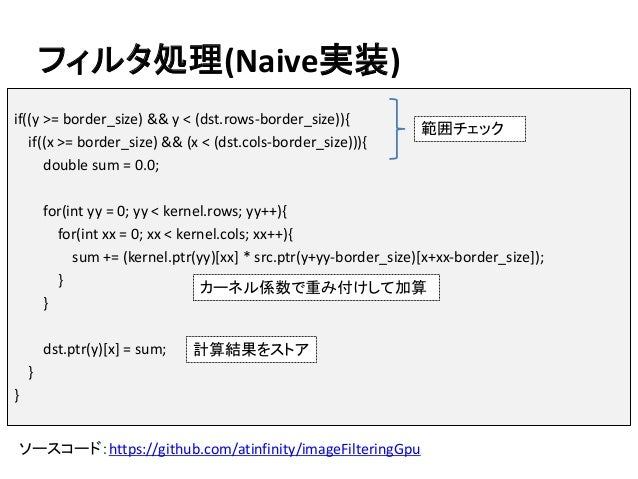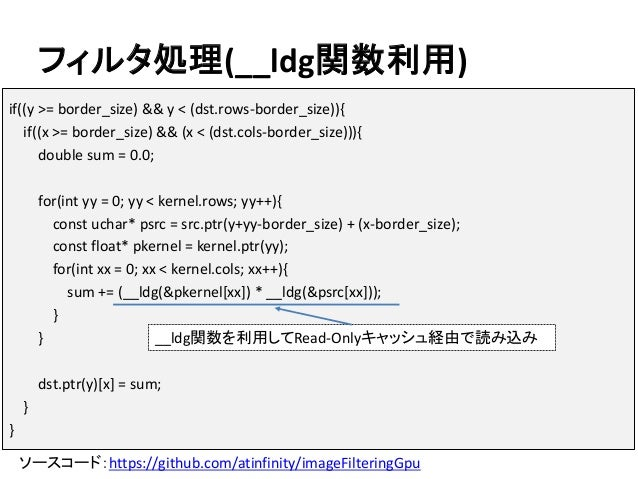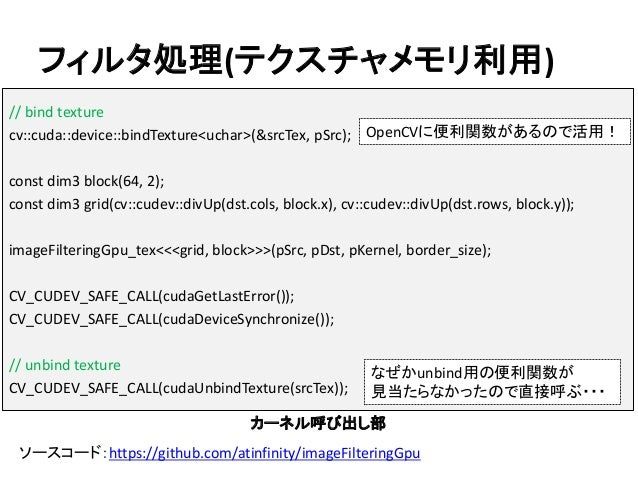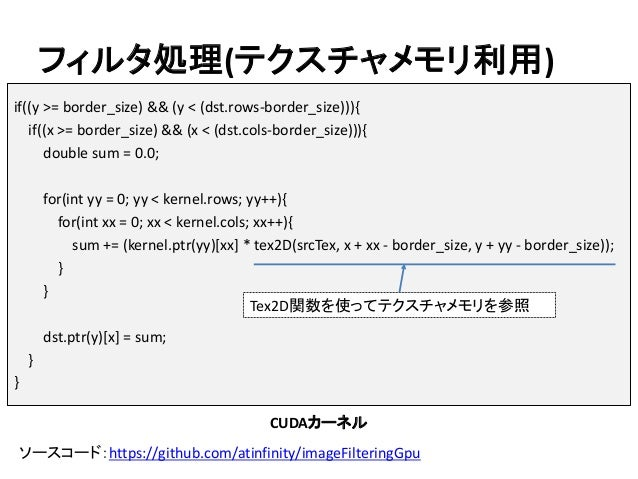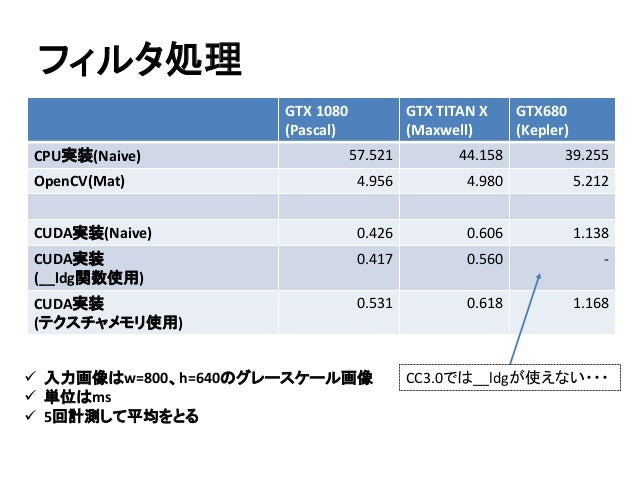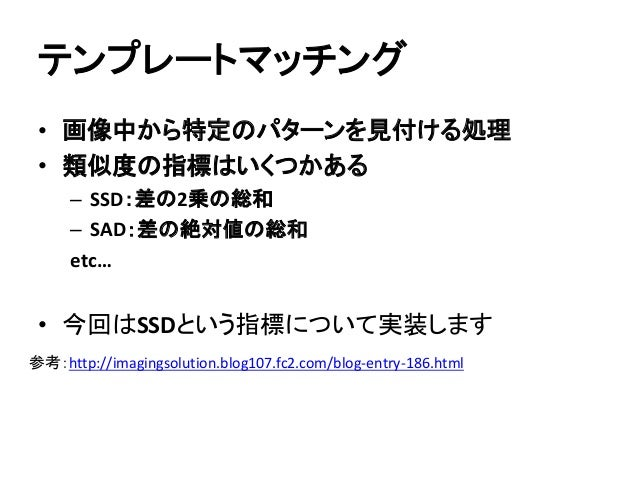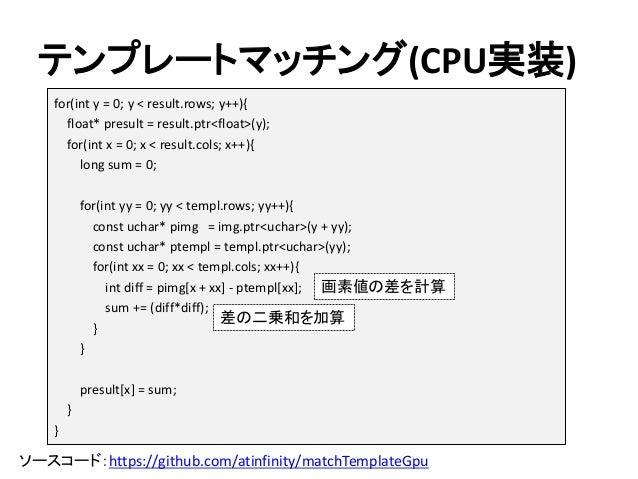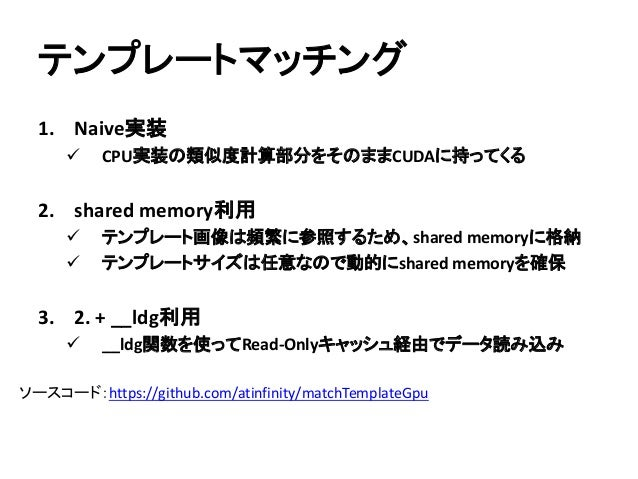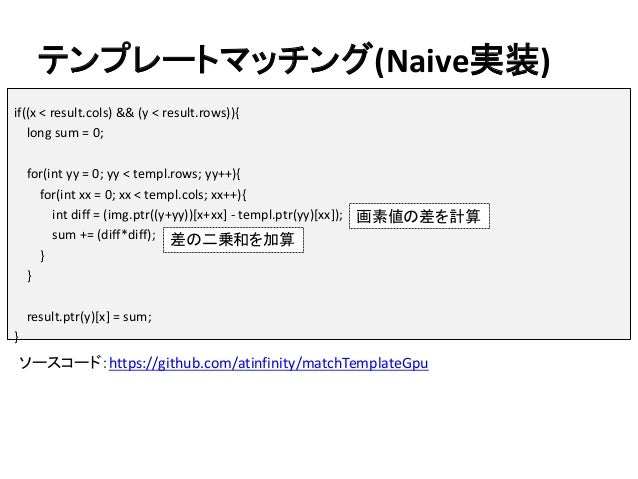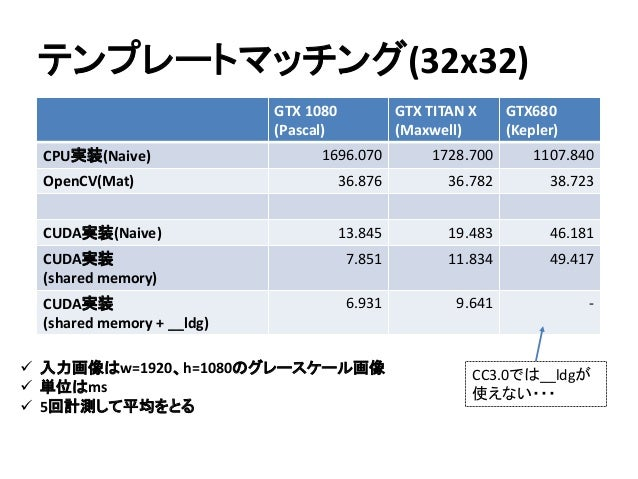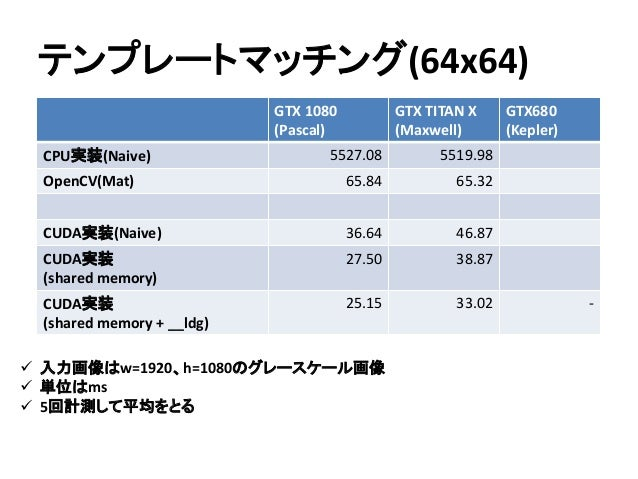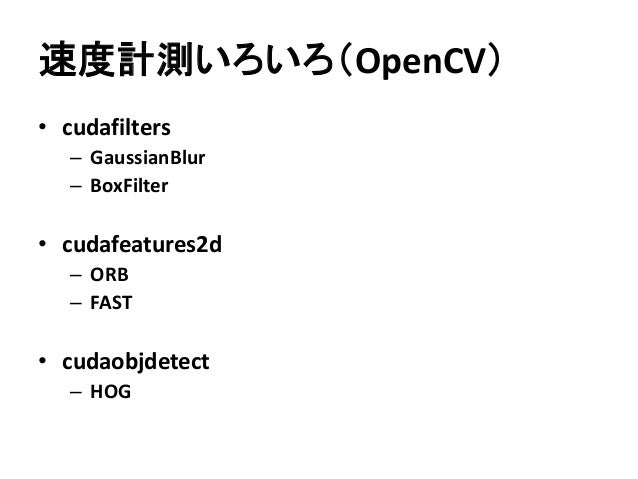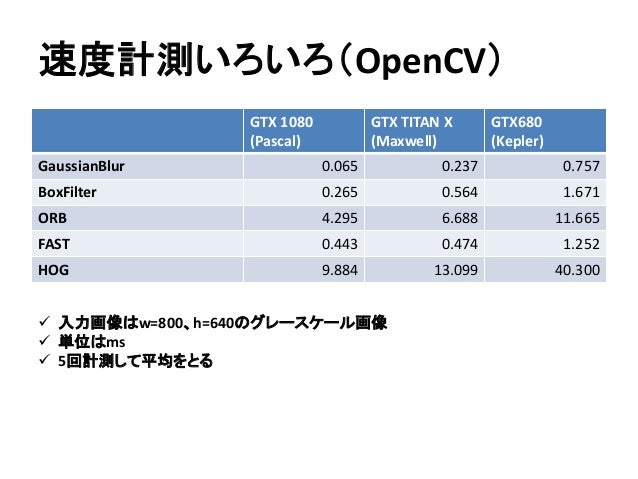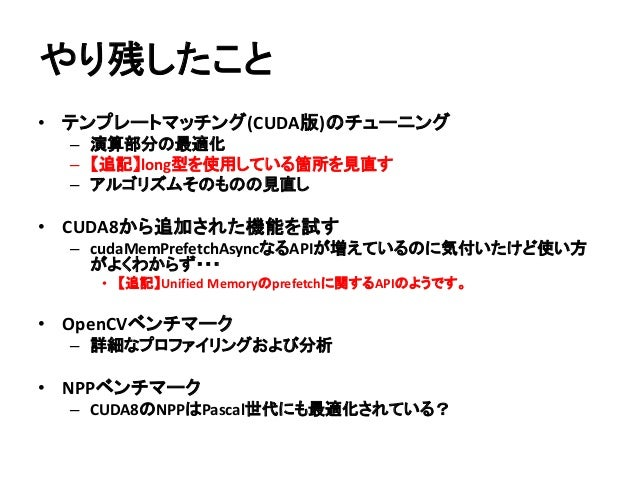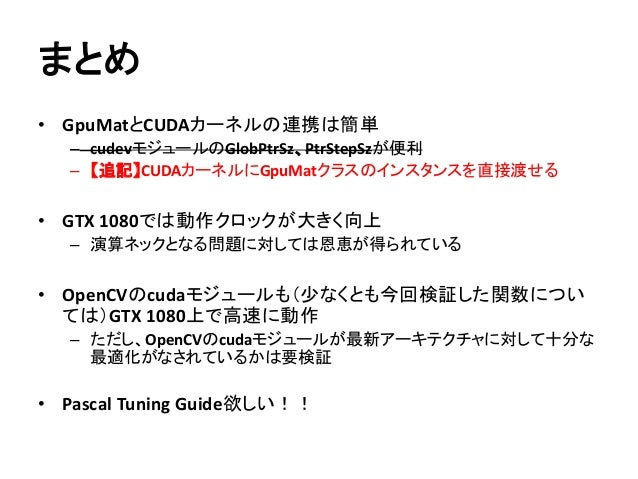Upcoming SlideShare
×
• Full Name
Comment goes here.

Are you sure you want to Yes No• Be the first to comment

### 【関東GPGPU勉強会#4】GTX 1080でComputer Vision アルゴリズムを色々動かしてみる

1. 1. GTX 1080でComputer Vision アルゴリズムを色々動かしてみる 関東GPGPU勉強会#4 2016/8/21 @dandelion1124
2. 2. 自己紹介(1/2) Twitter ID：@dandelion1124 • 学生時代はコンピュータビジョン、VRの研究に従事 • 大学院の研究室でOpenCVのTipsサイトを作っていたら OpenCV関連書籍（OpenCVプログラミングブック）を書くことに • 現在は都内勤務エンジニア Web: http://atinfinity.github.io Wiki: https://github.com/atinfinity/lab/wiki 連載記事: http://www.buildinsider.net/small/opencv/
3. 3. 自己紹介(2/2) • メインで参加している勉強会 – 関東コンピュータビジョン勉強会 #cvsaisentan http://sites.google.com/site/cvsaisentan/
4. 4. 本日のアジェンダ • OpenCVとは • OpenCVのデータ構造 • GpuMatとCUDAカーネルの連携 • CUDAカーネル実装 – フィルタ処理 – テンプレートマッチング • 速度計測いろいろ – OpenCV
5. 5. OpenCVとは Intelが開発したOpen SourceのComputer Vision ライブラリ。現在はItseezIntelが開発を行っている。 http://opencv.org/ • 公式サポートOS – Windows/Linux/Mac OS/Android/iOS • 公式サポート言語 – C/C++/Python/Java ※有志による非公式ラッパーの一覧を以下のサイトにまとめています。 https://github.com/atinfinity/lab/wiki/OpenCV-binding%E3%81%BE%E3%81%A8%E3%82%81 先日ItseezがIntelに買収される という発表がありました
6. 6. OpenCVのデータ構造 OpenCVで画像を格納するために使うデータ構造は おおまかに以下の4つ。 • Mat 画像データの入れ物（CPU版） • UMat 画像データの入れ物（CPU/OpenCL版） • GpuMat 画像データの入れ物（CUDA版） UMatについては前回勉強会の発表資料を参照ください。 http://www.slideshare.net/YasuhiroYoshimura/gpgpu-dandelion1124-201301130 ※上記資料は2013年11月時点のものなので最新版ではAPI仕様が少し変わっています。
7. 7. GpuMatとCUDAカーネルの連携 GpuMatとCUDAカーネルを連携する場合は、 cudevモジュールのGlobPtrSz、PtrStepSzを活用すると 便利です。 • GlobPtrSz：cols(幅)、rows (高さ) 、step等が参照可 • PtrStepSz：cols (幅)、rows (高さ)、step、ptr等が参照可 – Ptrメソッドを使うとポインタのアドレス計算を簡略化できる – 以降のコードではこちらを使います 参考：http://proc-cpuinfo.fixstars.com/2016/08/gpumat.html
8. 8. サンプルコード(PtrStepSz) // PtrStepSz型の変数を生成 cv::cudev::PtrStepSz<uchar> pSrc = cv::cudev::PtrStepSz<uchar>(src.rows, src.cols * src.channels(), src.ptr<uchar>(), src.step); const dim3 block(64, 2); const dim3 grid(cv::cudev::divUp(dst.cols, block.x), cv::cudev::divUp(dst.rows, block.y)); // CUDAカーネルを呼び出す kernel<<<grid, block>>>(pSrc); // カーネル引数としてPtrStepSz型の変数を渡す CV_CUDEV_SAFE_CALL(cudaGetLastError()); CV_CUDEV_SAFE_CALL(cudaDeviceSynchronize()); カーネル呼び出し部
9. 9. サンプルコード(PtrStepSz) // カーネル引数としてPtrStepSz型の変数を受け取る __global__ void kernel(cv::cudev::PtrStepSz<uchar> src) { int x = blockDim.x * blockIdx.x + threadIdx.x; int y = blockDim.y * blockIdx.y + threadIdx.y; if(x < src.cols && y < src.rows) { uchar val = src.ptr(y)[x]; // 座標(x, y)の画素値を参照する } } CUDAカーネル
10. 10. 【追記】GpuMatとCUDAカーネルの連携 GpuMatとCUDAカーネルを連携する場合は、 cudevモジュールのGlobPtrSz、PtrStepSzを活用すると 便利です。 発表時に「CUDAカーネルの引数としてGpuMatクラスの インスタンスを直接渡せる」というご指摘を受けたため、 確認したところ、正しく動作することがわかりました。 実装する際にはGpuMatクラスのインスタンスを渡した方が わかりやすそうです。
11. 11. 【追記】サンプルコード(GpuMat) cv::Mat src = cv::imread("lena.jpg", cv::IMREAD_GRAYSCALE); cv::cuda::GpuMat d_src(src); const dim3 block(64, 2); const dim3 grid(cv::cudev::divUp(dst.cols, block.x), cv::cudev::divUp(dst.rows, block.y)); // CUDAカーネルを呼び出す kernel<<<grid, block>>>(d_src); // カーネル引数としてGpuMatクラスのインスタンスを渡す CV_CUDEV_SAFE_CALL(cudaGetLastError()); CV_CUDEV_SAFE_CALL(cudaDeviceSynchronize()); カーネル呼び出し部
12. 12. 【追記】サンプルコード(GpuMat) // カーネル引数としてGpuMatクラスのインスタンスを受け取る __global__ void kernel(cv::cuda::GpuMat src) { int x = blockDim.x * blockIdx.x + threadIdx.x; int y = blockDim.y * blockIdx.y + threadIdx.y; if(x < src.cols && y < src.rows) { uchar val = src.ptr(y)[x]; // 座標(x, y)の画素値を参照する } } CUDAカーネル
13. 13. CUDAカーネル実装 よーし、CUDAカーネル書くぞー！ →まずはCUDA8のドキュメントを読もう →Pascal Tuning Guideはまだない？ ※CUDA 8 RCのドキュメント
14. 14. ご清聴ありがとうございました
15. 15. CUDAカーネル実装 よーし、CUDAカーネル書くぞー！ →まずはCUDA8のドキュメントを読もう →Pascal Tuning Guideはまだない？ →今回は手探りでやってみましょう ※CUDA 8 RCのドキュメント
16. 16. CUDAカーネル実装 • 計測環境 – OS：Ubuntu 16.04 LTS(64bit) – CUDA：CUDA Toolkit v8.0 – GCC：5.4.0 – CPU：Intel Xeon CPU E5-2623 v3 @ 3.00GHz – メモリ：128GB – GPU：GeForce GTX 1080 • Pascalアーキテクチャ
17. 17. CUDAカーネル実装 • 計測環境（比較用） – OS：Ubuntu 16.04 LTS(64bit) – CUDA：CUDA Toolkit v8.0 – GCC：5.4.0 – CPU：Intel Xeon CPU E5-2623 v3 @ 3.00GHz – メモリ：128GB – GPU：GeForce GTX TITAN X • Maxwellアーキテクチャ
18. 18. CUDAカーネル実装 • 計測環境（比較用） – OS：Windows 10 Pro(64bit) – CUDA：CUDA Toolkit v7.5 – Visual Studio：Visual Studio 2013 Update5 – CPU：Intel Core i7-3930K @ 3.20GHz – メモリ：32GB – GPU：GeForce GTX 680 • Keplerアーキテクチャ
19. 19. CUDAカーネル実装 GeForce GTX 1080 GeForce GTX Titan X GeForce GTX 680 アーキテクチャ Pascal Maxwell Kepler CUDAコア数 2560基 3072基 1536基 定格クロック 1607MHz 1000MHz 1006MHz ブーストクロック 1733MHz 1075MHz 1058MHz メモリバス帯域幅 320GB/s 336.5GB/s 192.2GB/s メモリ容量 8GB 12GB 2GB  動作クロックが大きく向上  メモリバス帯域幅はGeForce Titan Xとほぼ同等  CUDAコア、メモリ容量がGTX 1080より多い
20. 20. フィルタ処理 • 着目座標および周辺の画素値にカーネル係数で重み付けし た値を計算する処理 • 以下の例だと・・・ (1/9)*11 + (1/9)*14 + (1/9)*11 + (1/9)*13 + (1/9)*10 + (1/9)*13 + (1/9)*11 + (1/9)*14 + (1/9)*11 = 12 カーネル(3x3) 11 13 11 14 13 14 11 10 11 入力画像 1/9 1/9 1/9 1/9 1/9 1/9 1/91/91/9 注目座標 12 出力画像 積和演算が多い！！ この例だと積：9回、和：9回
21. 21. フィルタ処理(CPU実装) ソースコード：https://github.com/atinfinity/imageFilteringGpu for(int y = border_size; y < (dst.rows - border_size); y++){ uchar* pdst = dst.ptr<uchar>(y); for(int x = border_size; x < (dst.cols - border_size); x++){ double sum = 0.0; for(int yy = 0; yy < kernel.rows; yy++){ for(int xx = 0; xx < kernel.cols; xx++){ sum += (kernel.ptr<float>(yy)[xx] * src.ptr<uchar>(y+yy-border_size)[x+xx-border_size]); } } pdst[x] = sum; } } 計算結果をストア カーネル係数で重み付けして加算
22. 22. フィルタ処理 • Naive実装 – CPU実装の類似度計算部分をそのままCUDAに持ってくる • __ldg関数利用 – __ldg関数を使ってRead-Onlyキャッシュ経由でデータ読 み込み • テクスチャメモリ利用 – 画像データをテクスチャメモリに格納し、カーネルで参照 ソースコード：https://github.com/atinfinity/imageFilteringGpu
23. 23. フィルタ処理(Naive実装) ソースコード：https://github.com/atinfinity/imageFilteringGpu if((y >= border_size) && y < (dst.rows-border_size)){ if((x >= border_size) && (x < (dst.cols-border_size))){ double sum = 0.0; for(int yy = 0; yy < kernel.rows; yy++){ for(int xx = 0; xx < kernel.cols; xx++){ sum += (kernel.ptr(yy)[xx] * src.ptr(y+yy-border_size)[x+xx-border_size]); } } dst.ptr(y)[x] = sum; } } 範囲チェック カーネル係数で重み付けして加算 計算結果をストア
24. 24. フィルタ処理(__ldg関数利用) ソースコード：https://github.com/atinfinity/imageFilteringGpu if((y >= border_size) && y < (dst.rows-border_size)){ if((x >= border_size) && (x < (dst.cols-border_size))){ double sum = 0.0; for(int yy = 0; yy < kernel.rows; yy++){ const uchar* psrc = src.ptr(y+yy-border_size) + (x-border_size); const float* pkernel = kernel.ptr(yy); for(int xx = 0; xx < kernel.cols; xx++){ sum += (__ldg(&pkernel[xx]) * __ldg(&psrc[xx])); } } dst.ptr(y)[x] = sum; } } __ldg関数を利用してRead-Onlyキャッシュ経由で読み込み
25. 25. フィルタ処理(テクスチャメモリ利用) ソースコード：https://github.com/atinfinity/imageFilteringGpu // bind texture cv::cuda::device::bindTexture<uchar>(&srcTex, pSrc); const dim3 block(64, 2); const dim3 grid(cv::cudev::divUp(dst.cols, block.x), cv::cudev::divUp(dst.rows, block.y)); imageFilteringGpu_tex<<<grid, block>>>(pSrc, pDst, pKernel, border_size); CV_CUDEV_SAFE_CALL(cudaGetLastError()); CV_CUDEV_SAFE_CALL(cudaDeviceSynchronize()); // unbind texture CV_CUDEV_SAFE_CALL(cudaUnbindTexture(srcTex)); カーネル呼び出し部 OpenCVに便利関数があるので活用！ なぜかunbind用の便利関数が 見当たらなかったので直接呼ぶ・・・
26. 26. フィルタ処理(テクスチャメモリ利用) ソースコード：https://github.com/atinfinity/imageFilteringGpu if((y >= border_size) && (y < (dst.rows-border_size))){ if((x >= border_size) && (x < (dst.cols-border_size))){ double sum = 0.0; for(int yy = 0; yy < kernel.rows; yy++){ for(int xx = 0; xx < kernel.cols; xx++){ sum += (kernel.ptr(yy)[xx] * tex2D(srcTex, x + xx - border_size, y + yy - border_size)); } } dst.ptr(y)[x] = sum; } } Tex2D関数を使ってテクスチャメモリを参照 CUDAカーネル
27. 27. フィルタ処理  入力画像はw=800、h=640のグレースケール画像  単位はms  5回計測して平均をとる GTX 1080 (Pascal) GTX TITAN X (Maxwell) GTX680 (Kepler) CPU実装(Naive) 57.521 44.158 39.255 OpenCV(Mat) 4.956 4.980 5.212 CUDA実装(Naive) 0.426 0.606 1.138 CUDA実装 (__ldg関数使用) 0.417 0.560 - CUDA実装 (テクスチャメモリ使用) 0.531 0.618 1.168 CC3.0では__ldgが使えない・・・
28. 28. テンプレートマッチング • 画像中から特定のパターンを見付ける処理 • 類似度の指標はいくつかある – SSD：差の2乗の総和 – SAD：差の絶対値の総和 etc… • 今回はSSDという指標について実装します 参考：http://imagingsolution.blog107.fc2.com/blog-entry-186.html
29. 29. テンプレートマッチング(CPU実装) ソースコード：https://github.com/atinfinity/matchTemplateGpu for(int y = 0; y < result.rows; y++){ float* presult = result.ptr<float>(y); for(int x = 0; x < result.cols; x++){ long sum = 0; for(int yy = 0; yy < templ.rows; yy++){ const uchar* pimg = img.ptr<uchar>(y + yy); const uchar* ptempl = templ.ptr<uchar>(yy); for(int xx = 0; xx < templ.cols; xx++){ int diff = pimg[x + xx] - ptempl[xx]; sum += (diff*diff); } } presult[x] = sum; } } 画素値の差を計算 差の二乗和を加算
30. 30. テンプレートマッチング 1. Naive実装  CPU実装の類似度計算部分をそのままCUDAに持ってくる 2. shared memory利用  テンプレート画像は頻繁に参照するため、shared memoryに格納  テンプレートサイズは任意なので動的にshared memoryを確保 3. 2. + __ldg利用  __ldg関数を使ってRead-Onlyキャッシュ経由でデータ読み込み ソースコード：https://github.com/atinfinity/matchTemplateGpu
31. 31. テンプレートマッチング(Naive実装) ソースコード：https://github.com/atinfinity/matchTemplateGpu if((x < result.cols) && (y < result.rows)){ long sum = 0; for(int yy = 0; yy < templ.rows; yy++){ for(int xx = 0; xx < templ.cols; xx++){ int diff = (img.ptr((y+yy))[x+xx] - templ.ptr(yy)[xx]); sum += (diff*diff); } } result.ptr(y)[x] = sum; } 差の二乗和を加算 画素値の差を計算
32. 32. テンプレートマッチング(32x32)  入力画像はw=1920、h=1080のグレースケール画像  単位はms  5回計測して平均をとる GTX 1080 (Pascal) GTX TITAN X (Maxwell) GTX680 (Kepler) CPU実装(Naive) 1696.070 1728.700 1107.840 OpenCV(Mat) 36.876 36.782 38.723 CUDA実装(Naive) 13.845 19.483 46.181 CUDA実装 (shared memory) 7.851 11.834 49.417 CUDA実装 (shared memory + __ldg) 6.931 9.641 - CC3.0では__ldgが 使えない・・・
33. 33. テンプレートマッチング(64x64)  入力画像はw=1920、h=1080のグレースケール画像  単位はms  5回計測して平均をとる GTX 1080 (Pascal) GTX TITAN X (Maxwell) GTX680 (Kepler) CPU実装(Naive) 5527.08 5519.98 OpenCV(Mat) 65.84 65.32 CUDA実装(Naive) 36.64 46.87 CUDA実装 (shared memory) 27.50 38.87 CUDA実装 (shared memory + __ldg) 25.15 33.02 -
34. 34. 速度計測いろいろ（OpenCV） • cudafilters – GaussianBlur – BoxFilter • cudafeatures2d – ORB – FAST • cudaobjdetect – HOG
35. 35. 速度計測いろいろ（OpenCV）  入力画像はw=800、h=640のグレースケール画像  単位はms  5回計測して平均をとる GTX 1080 (Pascal) GTX TITAN X (Maxwell) GTX680 (Kepler) GaussianBlur 0.065 0.237 0.757 BoxFilter 0.265 0.564 1.671 ORB 4.295 6.688 11.665 FAST 0.443 0.474 1.252 HOG 9.884 13.099 40.300
36. 36. やり残したこと • テンプレートマッチング(CUDA版)のチューニング – 演算部分の最適化 – 【追記】long型を使用している箇所を見直す – アルゴリズムそのものの見直し • CUDA8から追加された機能を試す – cudaMemPrefetchAsyncなるAPIが増えているのに気付いたけど使い方 がよくわからず・・・ • 【追記】Unified Memoryのprefetchに関するAPIのようです。 • OpenCVベンチマーク – 詳細なプロファイリングおよび分析 • NPPベンチマーク – CUDA8のNPPはPascal世代にも最適化されている？
37. 37. まとめ • GpuMatとCUDAカーネルの連携は簡単 – cudevモジュールのGlobPtrSz、PtrStepSzが便利 – 【追記】CUDAカーネルにGpuMatクラスのインスタンスを直接渡せる • GTX 1080では動作クロックが大きく向上 – 演算ネックとなる問題に対しては恩恵が得られている • OpenCVのcudaモジュールも（少なくとも今回検証した関数につい ては）GTX 1080上で高速に動作 – ただし、OpenCVのcudaモジュールが最新アーキテクチャに対して十分な 最適化がなされているかは要検証 • Pascal Tuning Guide欲しい！！
38. 38. おわり

### Be the first to comment

•#### tokoik

Aug. 21, 2016
•#### Ergamion

May. 25, 2020

Total views

4,971

On Slideshare

0

From embeds

0

Number of embeds

132

22

Shares

0